# How to Find Simple Percentages of a Number

How to Find Simple Percentages of a Number#### Rules for Finding Basic Percentages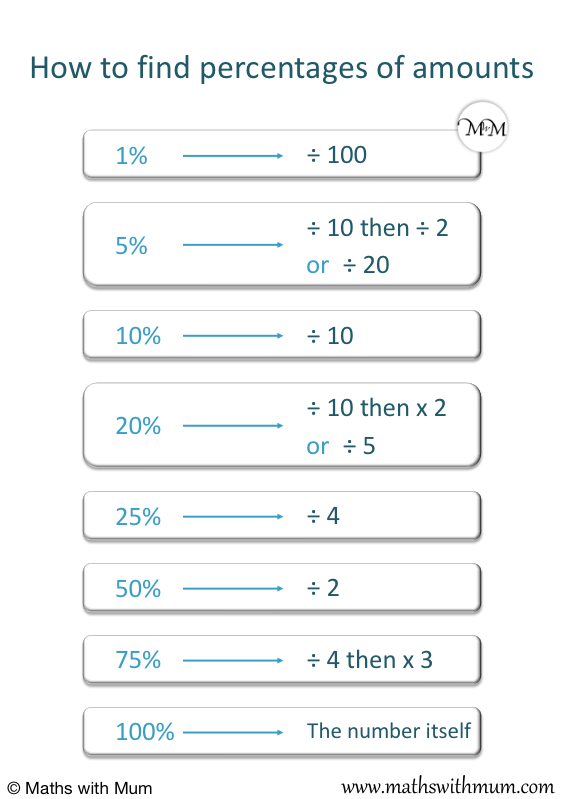• To find 1%, divide the number by 100.
• To find 5%, divide the number by 20 or find half of 10%.
• To find 10%, divide the number by 10.
• To find 20%, divide the number by 5 or find 10% and double it.
• To find 25%, divide the number by 4.
• To find 33.̄3%, divide the number by 3.
• To find 50%, divide the number by 2.
• To find 75%, divide the number by 4 and then multiply by 3.
Find simple percentages of a number by dividing using the rules shown above.• We want to find 20% of 40.
• To find 20% of a number, divide the number by 5 or find 10% and double it.
• 40 ÷ 5 = 8 and so, 20% of 40 = 8.
• Alternatively, we can find 10% and double it.
• To find 10% of 40, divide it by 10.
• 10% of 40 = 4.
• We double 10% to find that 20% = 8.Supporting Lessons

#### Finding Multiples of 10%# Simple Percentages of Amounts

## Rules for Finding Simple Percentages of a Number

The rules for finding simple percentages of a number are shown in the following table:

Percentage Rule
1% ÷ 100
5% ÷ 20
10% ÷ 10
20% ÷ 5
25% ÷ 4
33.̄3% ÷ 100
50% ÷ 2
75% ÷ 4 then × 3

To find the percentages of a number shown in the table above, simply divide the number using the rules provided.

Simple percentages are percentages of amounts that can be found with a simple division or multiplication. They do not require any addition of any smaller percentages.

For example, 50% is a simple percentage because we simply divide by 2 to find it. 31% is not a simple percentage we would need to calculate 30% and 1% and add them together.

Here is a poster showing how to calculate basic percentages.To introduce percentages, it is best to start with simple percentages and showing them as a fraction out of 100. For example, 50% means 50 out of 100, which is half. To find 50% of a number, simply half it.

Before looking at larger percentages, it is best to introduce basic percentages with a visual guide to how large they are. To teach how large a percentage is, you can show the percentage as a fraction of 100 squares.Start by introducing the following percentages first: 10%, 20%, 25%, 50%, 75% and 100%.

## How to Calculate 1% of a Number

To calculate 1% of a number, divide the number by 100. To divide by 100, move the decimal point two places left. For example, 1% of 135.6 = 1.356.

1% means 1 out of 100. 1% is the same as the fraction   1/100  .

To teach 1%, we can show 1 square shaded out of 100 squares.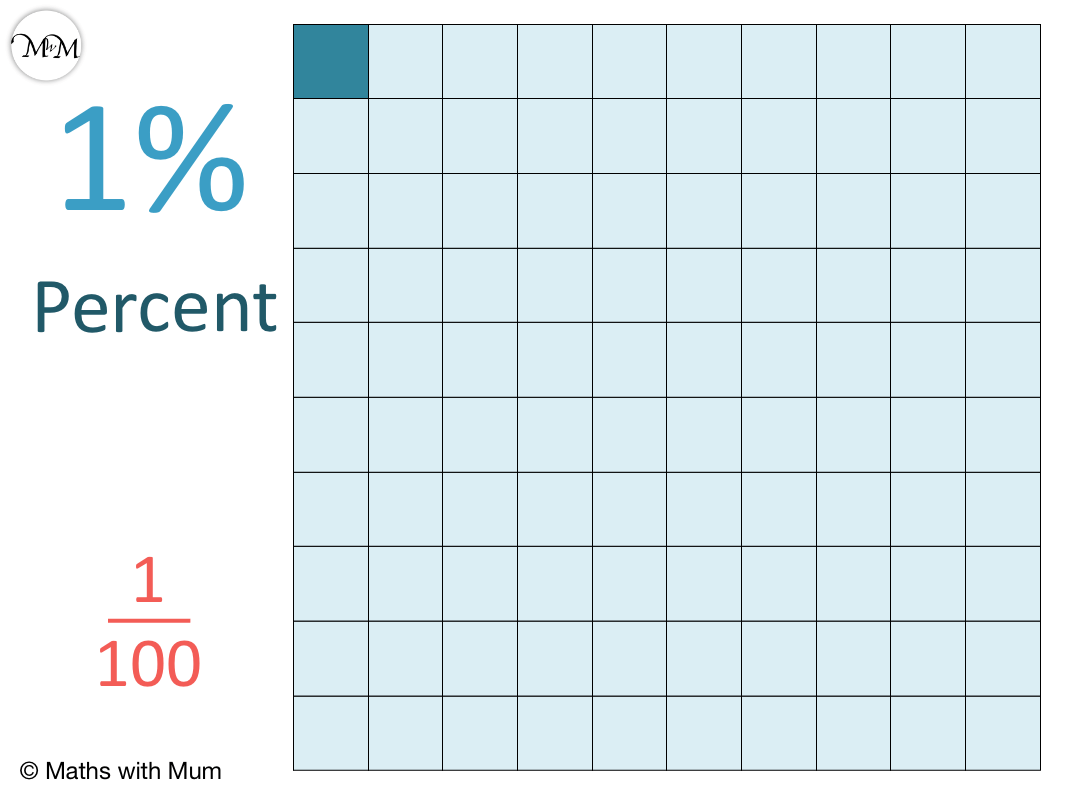1% means   1/100   and so, finding 1% of a number means to find   1/100   of that number.

To find 1% of a number without a calculator, divide the number by 100. The easiest way to do this is to move the decimal point 2 places to the left.

Here are some examples of finding 1%,

1% of 300 3
1% of 150 1.5
1% of 25 0.25
1% of 8 0.08
1% of 3.6 0.036
1% of 0.205 0.00205

To find 1% of a number with a calculator, divide the number by 100. You can also multiply the number by 0.01.

1% is a basic percentage because it can be found with one simple division. 1% is a very useful percentage to know how to find because you can use it to find any larger, whole number percentage of a number. Simply multiply the percentage required by the value of 1%.

## How to Calculate 5% of a Number

To find 5% of a number, divide the number by 20. This can be done by dividing the number by 10 and then halving it. For example, 5% of 40 = 2.

5% means 5 out of 100. 5% is the same as the fraction   5/100  , which can be simplified to   1/20  .

5% is a twentieth of the whole number.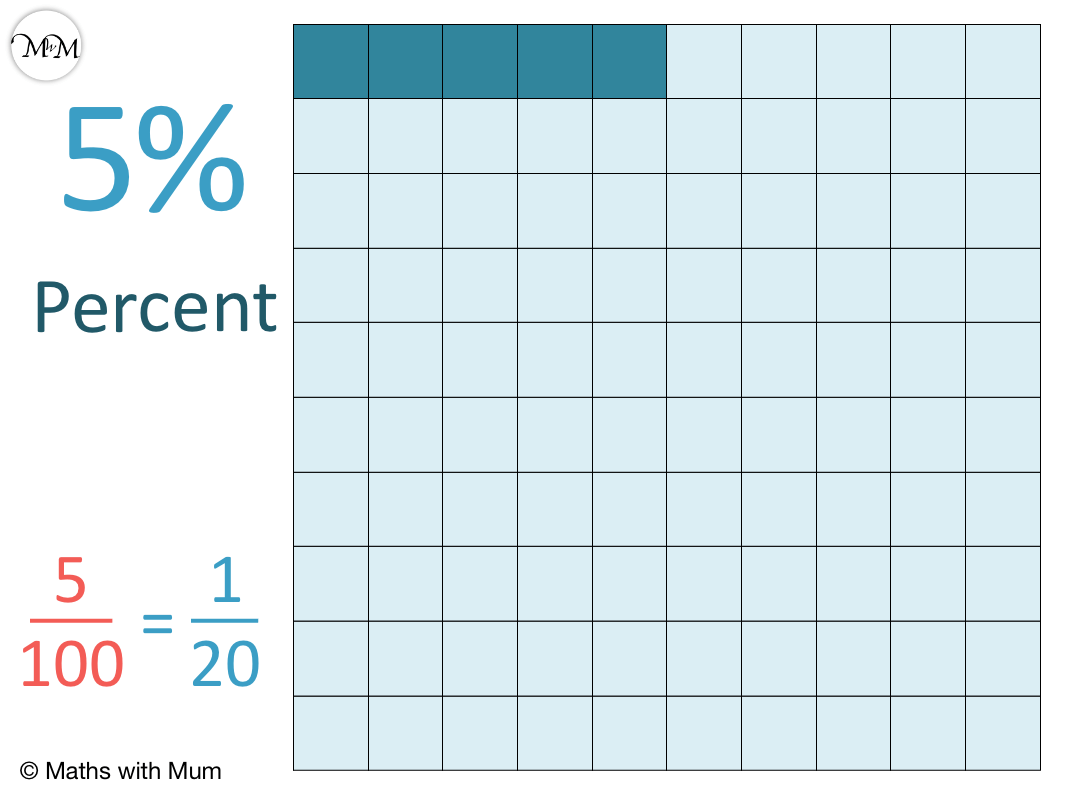5% means   1/20   and so, finding 5% of a number means to find   1/20   of that number.

To find 5% of a number without a calculator, divide the number by 20. The easiest way to do this is to divide by 10 and then halve the result.

Here are some examples of finding 5%,

5% of 200 10
5% of 60 3
5% of 30 1.5
5% of 8 0.4
5% of 1.6 0.08
5% of 0.402 0.0201

To find 5% of a number with a calculator, divide the number by 20. You can also multiply the number by 0.05.

Finding 5% is best to introduce after you have already learnt how to find 10%.

## How to Calculate 10% of a Number

To calculate 10% of a number, divide the number by 10. The easiest way to do this is to move the decimal point one place to the left. For example, 10% of 14.5 = 1.45.

10% means 10 out of 100. 10% is the same as the fraction   10/100  , which simplfies to   1/10  .

To teach 10%, we can show 10 squares shaded out of 100 squares.10% means   1/10   and so, finding 10% of a number means to find   1/10   of that number.

To find 10% of a number without a calculator, divide the number by 10. The easiest way to do this is to move the decimal point 1 place to the left.

Here are some examples of finding 10%,

10% of 1200 120
10% of 600 60
10% of 50 5
10% of 9 0.9
10% of 5.3 0.53
10% of 0.105 0.0105

To find 10% of a number with a calculator, divide the number by 10. You can also multiply the number by 0.1.

10% is a basic percentage because it can be found with one simple division. 10% is a very useful percentage to know how to find because we can easily multiply 10% to find other multiples of 10%, such as 20%, 30% etc.

## Multiples of 10% of a Number

To find multiples of 10% of a number, use these rules:

Percentage Rule
10% ÷ 10
20% ÷ 10 then × 2
30% ÷ 10 then × 3
40% ÷ 10 then × 4
50% ÷ 10 then × 5
60% ÷ 10 then × 6
70% ÷ 10 then × 7
80% ÷ 10 then × 8
90% ÷ 10 then × 9

Here is a poster showing how to find all of the multiples of 10% of a number.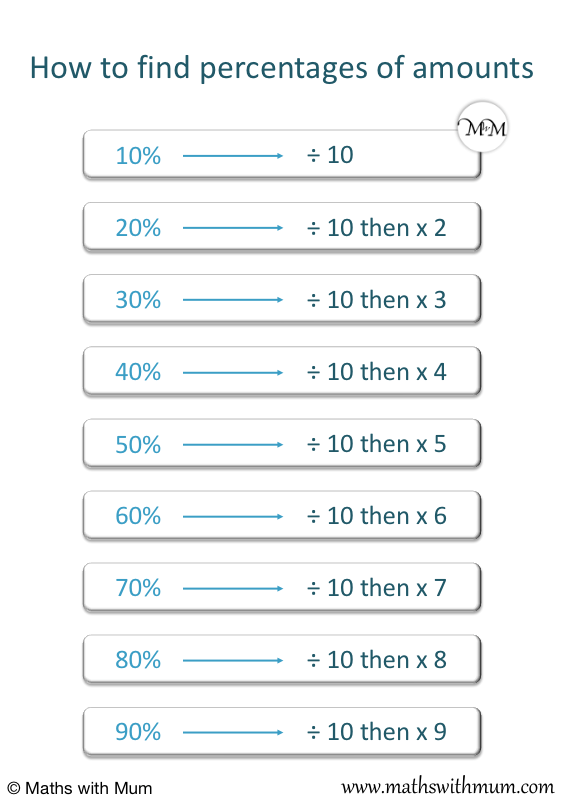For example, to find 30% of 50, divide 50 by 10 to find 10% and then multiply this by 3 to get 30%.To find 10% of 50, divide it by 10. 10% of 50 = 5.

Now that we have 10%, we simply multiply it by 3 to find 30%. 30% of 50 = 15.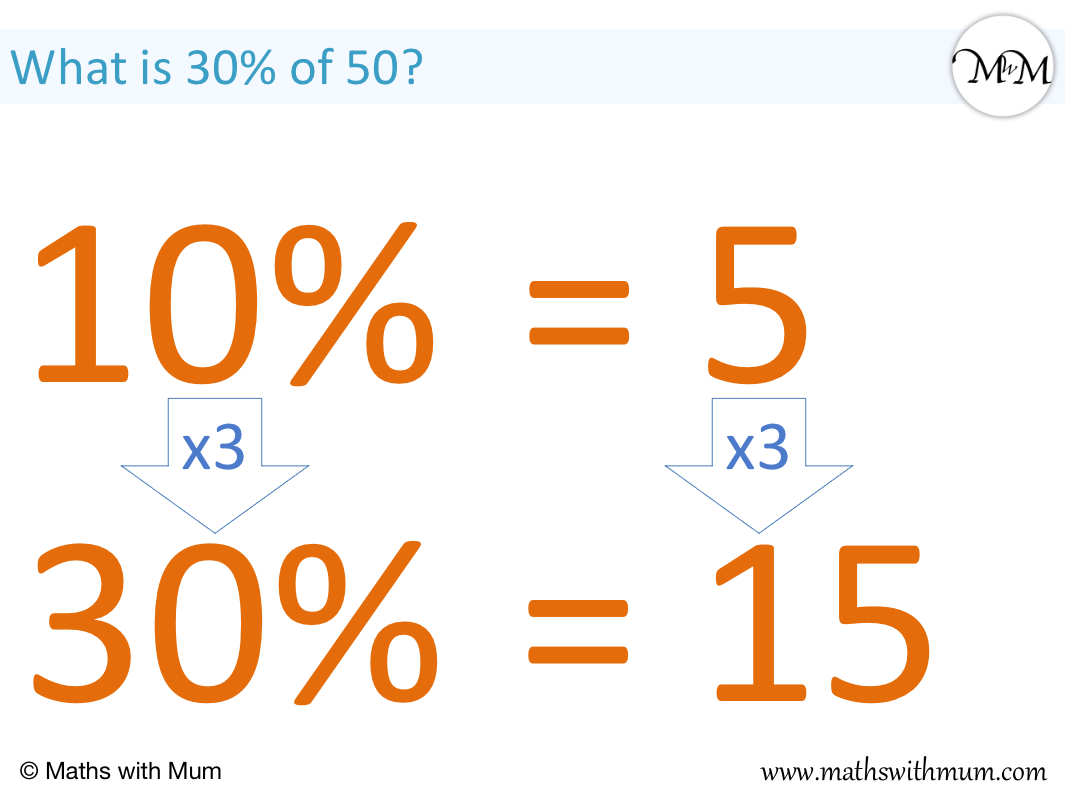Here is another example of finding a multiple of 10 percent. What is 40% of 70 grams?

To find 40% of a number, divide it by 10 to find 10% and then multiply this by 4.10% of 70 grams = 7 grams.

To find 40%, we multiply 10% by 4. 40% of 70 grams = 28 grams.Here we will find 80% of 120.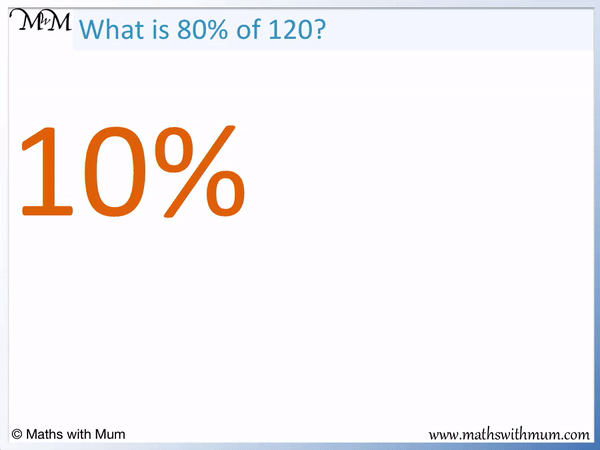To calculate 80% of a number, divide the number by 10 to get 10% and then multiply this by 8.

10% of 120 = 12.

80% is 10% multiplied by 8. 80% = 96, therefore 80% of 120 = 96.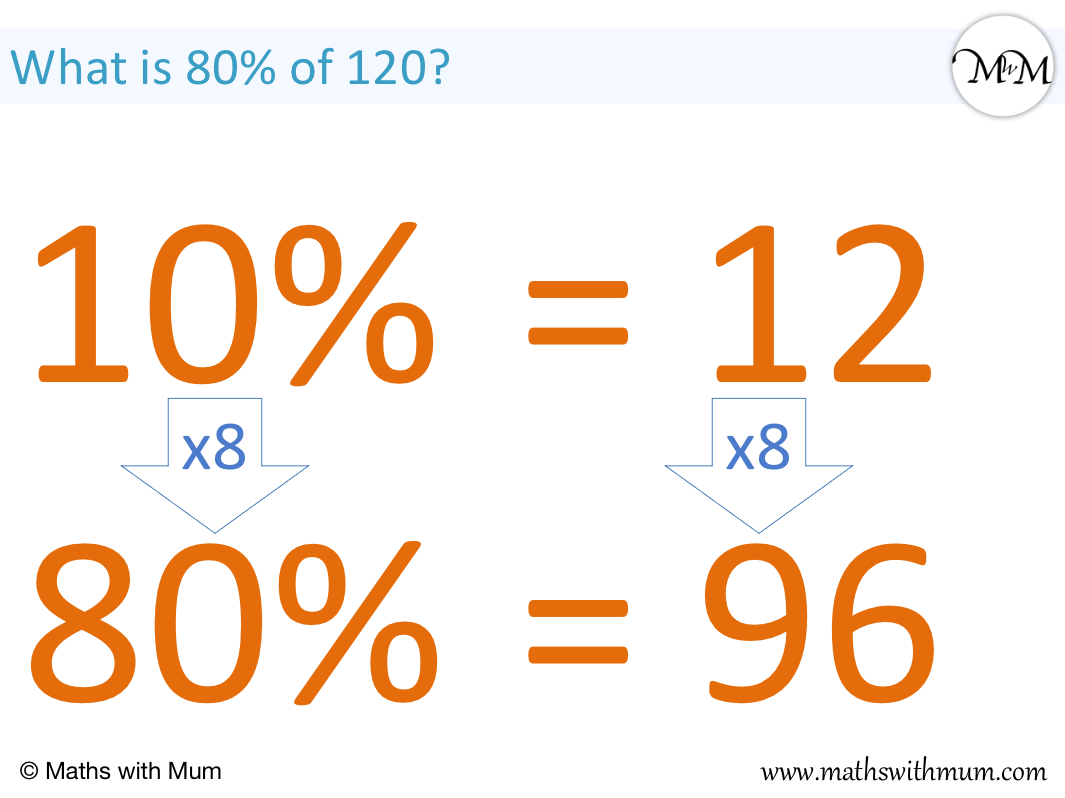## How to Calculate 20% of a Number

To calculate 20% of a number, divide it by 5. Alternatively, divide the number by 10 and then double it. For example, 20% of 30 = 6.

20% means 20 out of 100. 20% is the same as the fraction   20/100  , which can be simplified to   1/5  .

20% is a fifth of the whole number.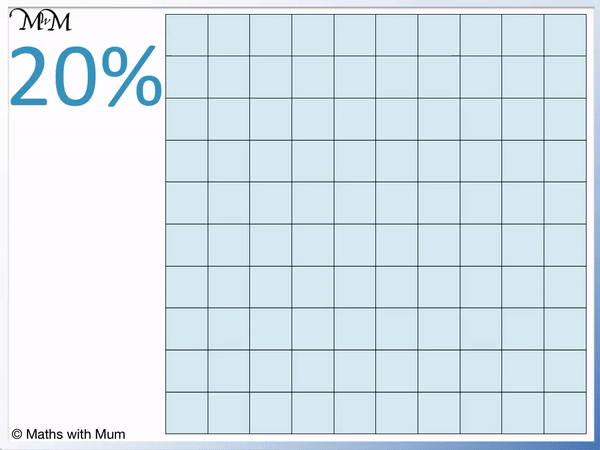20% means   1/5   and so, finding 20% of a number means to find   1/5   of that number.

To find 20% of a number without a calculator, divide the number by 5. Alternatively, divide the number by 10 and then double it.

Here are some examples of finding 20%,

20% of 400 80
20% of 20 4
20% of 70 14
20% of 5 1
20% of 6.1 1.22
20% of 0.303 0.0606

To find 20% of a number with a calculator, divide the number by 5. You can also multiply the number by 0.2.

## How to Calculate 33   1/3   % of a Number

To calculate 33   1/3   % of a number, divide it by 3.

3   1/3   is the same as 33.̄3%. To find 3   1/3   or 33.̄3%, divide the number by 3.33.̄3% means   1/3   and so, finding 33.̄3% of a number means to find   1/3   of that number.

To find 33.̄3% of a number without a calculator, divide the number by 3.

To find 33.̄3% of a number with a calculator, divide the number by 3. You can also multiply the number by 0.̄3.

## How to Calculate 50% of a Number

To calculate 50% of a number, divide the number by 2. For example, 50% of 20 = 10.

50% means 50 out of 100. 50% is the same as the fraction   50/100  , which simplfies to   1/2  .

To teach 50%, we can show 50 squares shaded out of 100 squares.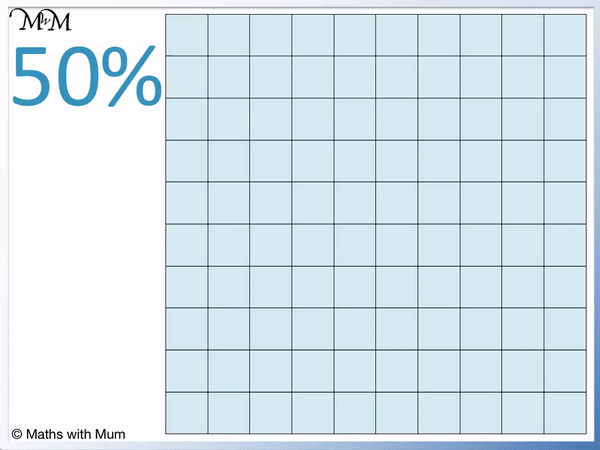50% means   1/2   and so, finding 50% of a number means to find   1/2   of that number.

To find 50% of a number without a calculator, divide the number by 2. To divide an odd number by 2, subtract one, half it and then add 0.5

Here are some examples of finding 50%,

50% of 800 400
50% of 300 150
50% of 20 10
50% of 7 3.5
50% of 2.6 1.3
50% of 0.804 0.402

To find 50% of a number with a calculator, divide the number by 2. You can also multiply the number by 0.5.

## How to Calculate 75% of a Number

To calculate 75% of a number, divide the number by 4 and then multiply this result by 3. For example 75% of 20 = 15.

75% means 75 out of 100. 75% is the same as the fraction   75/100  , which simplfies to   3/4  .

75% is three quarters of an amount.

To teach 75%, we can show 75 squares shaded out of 100 squares. It is helpful to shade the squares in the four corners as shown.75% means   3/4   and so, finding 75% of a number means to find   3/4   of that number.

To find 75% of a number without a calculator, divide the number by 4 and then multiply by 3.

Here are some examples of finding 75%,

75% of 800 600
75% of 300 225
75% of 60 45
75% of 4 3
75% of 6.8 5.1
75% of 0.334 0.2505

To find 75% of a number with a calculator, divide the number by 4 and then multiply by 3. You can also multiply the number by 0.75.

## What is 100% of a Number?

100% of a number is the whole of the number. 100% means one whole and so, 100% of a number is simply the number itself.

100% means 100 out of 100. 100% is the same as the fraction   100/100  , which simplfies to 1 whole.

We can see in this diagram that 100% shows all 100 squares shaded in.To find 100% of something simply means to find the whole amount of it. For example, 100% of 25 = 25.Now try our lesson on How to Calculate Percentages of Numbers where we learn how to find any percentage of a number.